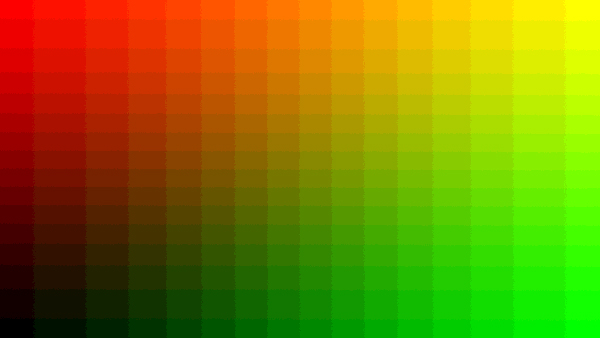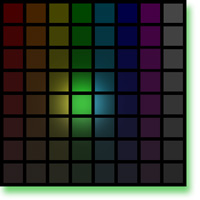# Numbers (Level 5)In this image, only sixteen numbers are used to represent various amounts of red (R) or green (G) and all their possible combinations. The lower left (black) rectangle shows R=0 and G=0. The upper right (bright yellow) shows R=15 and G = 15. The upper left is all red (R=15) with no green (G=0) and the lower right is all green (G=15) with no red (R=0). The rest of the rectangles show all possible combinations of 16 levels of red and 16 levels of green. You can think of this picture as a graph of color with the green value increasing along the horizontal (X) axis and red value increasing along the vertical (Y) axis. Instead of showing the numbers on this graph, we are seeing the colors produced on your display by those numbers. Throughout this image, blue is set to zero.

###### How Does a Computer Represent Colors as Numbers?

Computers represent everything as numbers. In fact, they represent everything using only two numbers, zero and one. Expressing numbers as zeroes and ones is known as using binary numbers. In computers the numbers are represented by electronic switches. An open switch represents a zero and a closed switch represents a one. With enough switches, virtually anything can be represented as numbers. Another name for these switches is "bits". These bits are stored in our computers' memories. Eight bits together makes what we call a "byte" of data. You've probably heard of how many megabytes (approximately millions of bytes) or gigabytes (approximately trillions of bytes) your computer can store. Ultimately the amount of memory in your computer determines how many numbers can be represented. Most commonly, colors are represented in computers using 8-bit numbers. This means that a set of eight zeroes and ones is used to represent a given color component. Every possible combination of eight zeroes and ones gives us 256 possible levels of color we can represent. For example the decimal integer 0 is represented in 8-bit binary digits as 00000000, while the decimal integer 255 is represented as 11111111.

There are many ways to represent colors with numbers. The most common method in computers is to represent the amount of red, green, and blue primary lights required to mix together to create the desired colors. This is the tradition because most computer displays work by adding together amounts of RGB primaries and the numbers can be used to directly display colors. If 8-bit numbers are used, then we can have values ranging from 0 - 255 for each of the RGB primaries of the color. In that case, black would be represented by (R=0,G=0,B=0) and white by (255,255,255). The red, green, and blue primaries would be represented by (255,0,0), (0,255,0), and (0,0,255) respectively. Similarly the cyan, magenta, and yellow secondaries would be represented by (0,255,255), (255,0,255), and (255,255,0). Intermediate colors are represented with intermediate numbers. For example a middle gray might be (128,128,128) and a pale yellow color (200,180,120).

As mentioned above, computers represent these numbers as binary numbers instead of decimal integers. Some computer programs represent colors in hexadecimal numbers. Hexadecimal doesn't have ten numerals like decimal (0123456789), but rather has 16 numerals represented by our normal decimal numerals and the first 6 letters of the alphabet (0123456789ABCDEF). The list below shows examples of colors represented with 8-bit decimal, binary, and hexadecimal numbers that all mean exactly the same thing.

Color Name: (Decimal RGB), (Binary RGB), (Hexadecimal RGB)
Black: (0,0,0), (00000000,00000000,00000000), (00,00,00)
White: (255,255,255), (11111111,11111111,11111111), (FF,FF,FF)
Red: (255,0,0), (11111111,00000000,00000000), (FF,00,00)
Green: (0,255,0), (00000000,11111111,00000000), (00,FF,00)
Blue: (0,0,255), (00000000,00000000,11111111), (00,00,FF)
Cyan: (0,255,255), (00000000,11111111,11111111), (00,FF,FF)
Magenta: (255,0,255), (11111111,00000000,11111111), (FF,00,FF)
Yellow: (255,255,0), (11111111,11111111,00000000), (FF,FF,00)
Gray: (128,128,128), (10000000,10000000,10000000), (80, 80, 80)
Pale Yellow: (200,180,120), (11001000,10110100,01111000), (C8,B4,78)

Ultimately it is the display or decoding of the numbers that determines the color that you see. (200,180,120) does not turn out to be exactly the same color on all computer displays or printers. This complexity is what makes accurate color reproduction a serious technical and scientific challenge.Explore the NEXT TOPIC at this level.

Explore the NEXT LEVEL on this topic.

Updated: Apr. 19, 2011